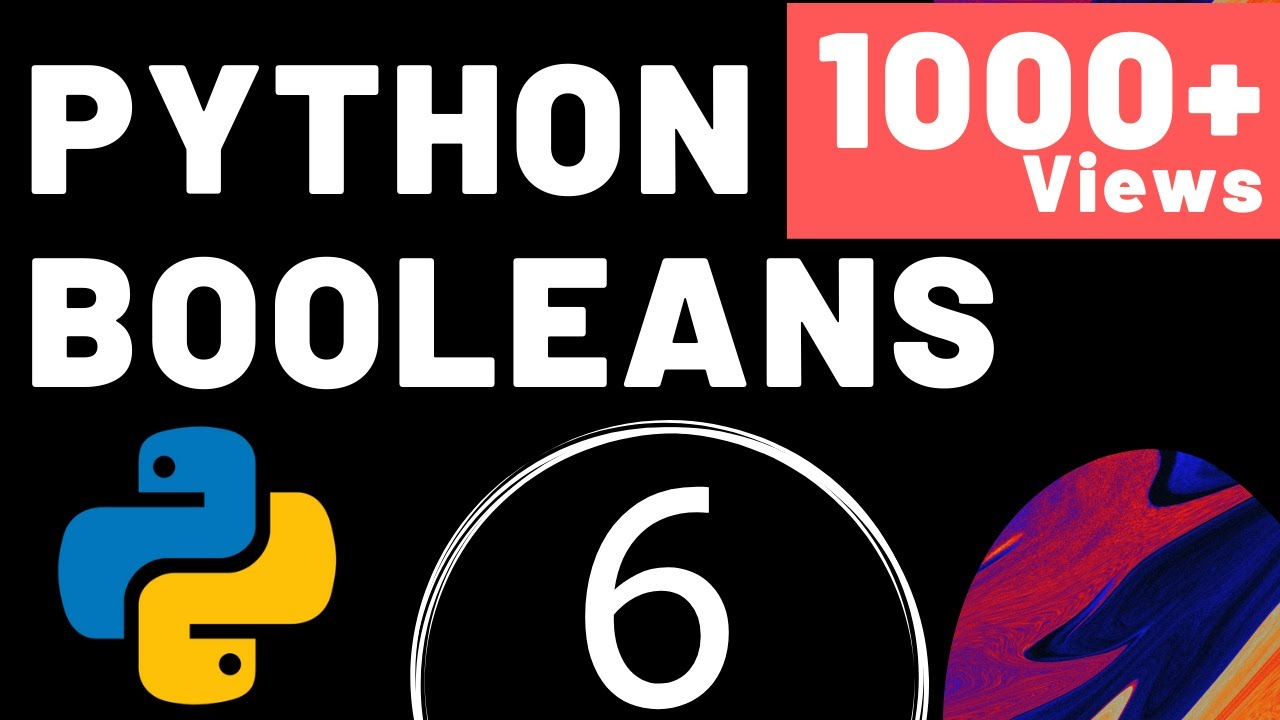Công Nghệ

# Booleans in Python in Hindi | Lecture 6 | Python Tutorial | Mayank Gupta#Programming #Python #Booleans
This Python Tutorial by Mayank Gupta, you will learn about Boolean Operators in Python, Equality and Non-Equality Operator, Comparison Operators, ‘or’, ‘and’, ‘not’ Operators and at last Test Your Skills.

Today’s Subtopics :
1. What is Boolean?
2. Equality and Non-Equality Operator.
3. Comparison Operators
4. ‘or’, ‘and’, ‘not’ Operators
5. Test your skills

1. What is Boolean?
Boolean Operators are simple words (AND, OR, NOT) used as conjunctions to combine or exclude keywords in a search, resulting in more focused and productive results.
The output will always be in True or False.

2. Equality and Not Equality Operator
i) ‘==’ Equality Operator :
If the values of two operands are equal, then the condition becomes true.
ii) ‘!=’ Not Equality Operator :
If the values of two operands are not equal, then the condition becomes true.

3. Comparison Operators
i ) Greater than Operator
If value of left operand is greater than the value of right operand, then condition becomes true.
ii ) Less than Operator
If the value of left operand is less than the value of right operand, then condition becomes true.
iii ) Greater than or Equal to Operator
If the value of left operand is greater than or equal to the value of right operand, then condition becomes true.
iv ) Less than or Equal to Operator
If the value of left operand is less than or equal to the value of right operand, then condition becomes true.

4. ‘or’, ‘and’, ‘not’ Operator
i ) ‘or’ Operator
Outputs True if either of the operands is true.
ii ) ‘and’ Operator
Outputs False if either of the operands is False.
iii ) ‘not’ Operator
Outputs True if operand is false. It complements the operand.

Test Your Skills :
x = 10
y = 20
z = 30
print(x (greater than symbol) 20 and y (greater than symbol) 30 and z (less than symbol) 50)
print(x (greater than symbol) 20 or y (greater than symbol) 30 or z (less than symbol) 50

If you liked the video, please Like, Comment and share and don’t forget to Subscribe my Channel.

Thank You
Mayank Gupta
#Python_In_Hindi

Nguồn: https://truonggiabinh.com

Xem thêm bài viết khác: https://truonggiabinh.com/cong-nghe/

Previous article

Next article

## 2 Comments

1.vaibhav chaudhary says:

False
True
Thankyou sir 🙏

2.Faiz Alam says:

False
True